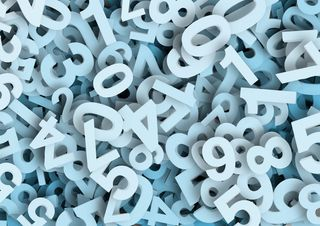Verified by Psychology Today# Are Your Number Coincidences Significant?

## Use this test to decide if you're seeing a certain number more than chance.Source: geralt/Pixabay

Of all the kinds of coincidences that people routinely experience, one of the most common is number coincidences. This isn’t surprising, since we are constantly surrounded by numbers, from dates to times to prices. But the fact that we are swimming in a sea of numbers does make it more difficult to determine whether, when we notice a particular number cropping up again and again, it really is occurring more often than would be expected by chance, or whether we’re just being unconsciously selective in what we pay attention to.

Fortunately, there are ways of determining whether a certain number really is appearing at a rate above chance. If you’re curious to find out about the significance of the number coincidences in your own life, you may want to try a little experiment I call the “License Plate Test.”

This test is based on keeping track of how many license plates you see and how many of them display numbers that end with the particular number you’re interested in.

### Preparation

Before beginning the test, you should write down the number you’re going to test for, which we’ll call your “target number.”

Next, you need to determine how often the numbers on license plates would end with your target number purely by chance. If your target number has two digits, then on one in every 100 plates that display a number at least two digits long, your target number will end the numerical portion of the plate just by chance. We can express the rate of 1 in 100 as 0.01. If your target number has three digits, this number will go down to 1 in every 1000 plates (of those that display numbers at least three digits long), a rate of 0.001. And if your target number has four digits, the rate of occurrence (on plates with at least four numerical digits) will be 0.0001. Let's call the rate that you calculate for your particular case your "chance baseline rate."

Now you need to write down one more number: how many total license plates you are going to observe for your experiment. It’s important to choose this number ahead of time, so that the stopping point of your experiment isn’t influenced by the results you’ve accumulated up to that point. Once you’ve observed your predetermined number of license plates—what we’ll call your “stopping number"—the experiment will be over.

### Gathering Data

Once you've written down all of this preliminary information, you can start gathering data. You will need to keep two running totals: (1) how many license plates you see that have at least the number of numerical digits that your target number has and (2) how many times the numerical portion of these plates ends with your target number.

For example, if your target number is 417, you're going to keep track of the total number of plates you see that have a number with at least three digits, and you're also going to keep track of how many of these numbers end in 417.

### Calculating Results

Once you reach your stopping number of total plates observed, it’s time to stop gathering data and calculate the significance of your results. If you divide your number of sightings of your target number by your total number of plates observed, you’ll immediately be able to see whether your actual rate of sightings exceeded the chance baseline rate.

However, it’s important to realize that the chance baseline rate can often be exceeded just by chance, and not because anything spooky is going on. With random processes being random, the number of sightings will always vary somewhat, sometimes exceeding the chance baseline rate and sometimes falling below it.

If your rate of sightings exceeds your chance baseline rate and you want to know what the precise odds are that you would get such a high number of sightings of your target number purely by chance, one way to do this is by using a binomial probability calculator, such as the one available for free at this link. This calculator will ask you for three numbers:

• “Probability of success on a single trial” is your chance baseline rate.
• "Number of trials" is your stopping number.
• "Number of successes (x)" is the number of sightings you had of your target number.

Using this information, the binomial probability calculator will tell you how likely it is, just on chance, that you would have observed your target number this many times or more over the course of your experiment. Actually, the calculator linked to above will return five different numbers. The one that tells you this is the last one: the cumulative probability labeled P(X ≥ x).

If this number turns out to be less than 0.05, then your test achieved what is commonly called “statistical significance.” Now it's up to you to figure out what the source of this potentially non-random pattern might be! It could be something as prosaic as an error in your data-gathering procedure or a bias in the way license plate numbers are distributed in your area. On the other hand, perhaps it is one of the intriguing phenomena explored in other posts on this blog. See, for example, my recent post "Mind Over Matter."